# AP Physics 1 Question 291: Answer and Explanation

### Test Information

Question: 291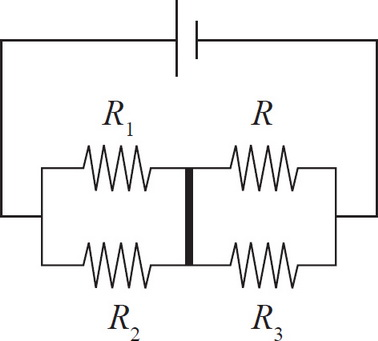9. In order to ensure that no current will pass through the cross path (bold in the image above), what must the resistance of R be in terms of R1, R2, and R3 ?

• A. R =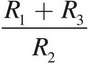• B. R =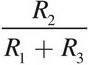• C. R =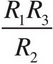• D. R =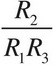In a circuit with multiple paths, the current through each path is inversely proportional to the resistance of that path. If no current passes through the indicated segment of wire, then the ratio (current in top path)/(current in bottom path) is the same before the cross bar as it is after the cross bar. Thus,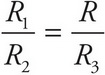, which means R =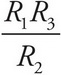.